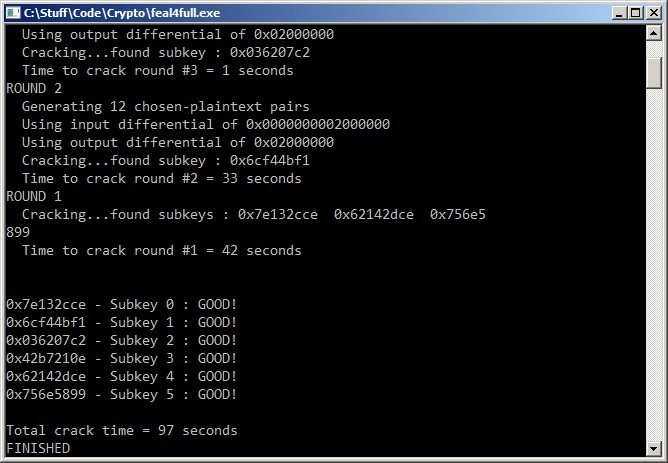# Differential Cryptanalysis of FEAL

Now, we'll take a deeper look at Feistel ciphers. I briefly mentioned these on the block ciphers page, but they deserve some more detail. This structure is used in a ton of modern block ciphers like DES, Blowfish, FEAL, and RC5. This architecture has a few advantages that make it attractive. For one, it has been studied a ton. The volume of work done on DES alone has lead to a lot of understanding of these structures in the academic crypto world. Also, it allows the core of the cipher to be a one-way function. In other words, the portion of a Feistel cipher that makes it secure does not need to be reversible. This is somewhat analogous to turning a hashing function into a cipher.

## Source Code

Here is the code I wrote that demonstrates the technique described on this page. Writing clear code that is easy to understand by humans is not a strong point of mine. Be sure that you have the diagrams on this page in front of you (preferably printed out) while reading the code. Enjoy!

FEAL-4 Differential Cryptanalysis Code - [feal4full.c]

### FEAL-4

The target throughout this tutorial will be FEAL-4. FEAL stands for Fast data Encipherment ALgorithm. This is the first "real world" cipher we've examined so far and it is somewhat unique in the crypto world. For one thing, it is notoriously vulnerable to just about every statistical attack out there. As a result, the FEAL family of block ciphers has become a whipping boy for cryptanalysts. For noobs like us, this makes it perfect for learning the ropes.

FEAL-4 is a 4 round Feistel cipher with a 64 bit block size. This means that the algorithm encrypts/decrypts data in 64 bit chunks. The Feistel structure means that the blocks are actually split in half for processing. These halves are mixed together via XOR operations throughout the encryption. The non-linear component (the heart of the cipher) is called the round function. It is a one-way/trapdoor function that takes a 32 bit input and produces a 32 bit output. This function is used 4 times during encryption: once for each round. The strength of FEAL-4 against statistical attacks like differential cryptanalysis is dependent on the behavior of this round function.

The 64 bit key is expanded to six 32 bit subkeys. The somewhat seperate process that achieves this is known as the key schedule. In the case of FEAL, this process is designed to be one-way. In other words, if you are able to recover one of the subkeys, it should be impossible to use that information to recover part of the "real" 64-bit key. Luckily, we only need all of the subkeys that are generated by the key schedule (or our own cryptanalysis efforts) to decrypt goodies. This means that recovering the original 64-bit key is not required. We'll ignore the key scheduling process for now and pretend that the six 32-bit subkeys are all generated randomly/independently. In other words, we are behaving as if FEAL-4 has a 192 bit key.

### Structure of FEALThe overall layout of FEAL is that of a Feistel cipher. It splits the data being encrypted into left and right halves. The right half is mixed with the round's subkey, then it is run through a one-way function called the round function (designated by f in the diagram) and combined with the left half using the XOR operation. The resulting halves are then swapped and the process is repeated for the other rounds. Before any of these rounds happen, subkeys 4 and 5 are used to XOR the incoming plaintext. This bookends the cipher and ensures that all 4 rounds actually matter.

The mixing of the subkeys using XOR is, by far, the gold standard for getting keying material into a cipher. The problem is that its very easy to "undo" XOR and recover the key if an attacker holds some plaintext and some ciphertext. To prevent this, cipher should employ elements of confusion and diffusion. In the case of FEAL, the round function provides both. Although there is some diffusion happening inherently in the Feistel structure itself (left and right halves mixing), most happens in the round function. As far as I can tell, the the overall structure does not provide confusion at all, but the round function does.

One way to sneak confusion/diffusion into a cipher is by doing substitution and permutation operations between rounds of key mixing. This technique is used in substitution-permutation networks (SPNs). These operations obscure the key mixing and make it impossible to easily trace your way back to the keys algebraically. There is a drawback, however; the substitutions (sboxes) and permutations (pboxes) must be reversible. This is necessary so that ciphertext can be decrypted back into plaintext. If the sboxes of an SPN were one-way functions, no one would be able to decrypt data produced by it. One-way functions are attractive because cipher designers can be more creative and produce better statistical properties with them. Feistel structures allow cryptographers to use a single one-way function for both encryption and decryption.

So, lets walk through the cipher...the plaintext enters ready for encryption:

Beginning: First, its left half is XOR'd with subkey 4 and its right half is XOR'd with subkey 5. Next, this new left half is XOR'd with this new right half to produce an even newer right half. The left half is just chillin' waiting for the right half to catch up. The right half is ready to continue with the next operation. The preparation is done now and this state is the beginning of a round of FEAL.

Each Round: The right half splits off and becomes the final left half for this round. But, we still need a final right half so lets do some work. The original right half is XOR'd with this round's subkey to produce an input for the round function. The round function processes this input and spits out a number. Remember when I said that the original left half was just chillin'? Well, its time to move, so he XORs with the number spit out by the round function. This XOR result becomes the final right half for this round. The next round of the cipher these left and right final halves and uses them for its input left and right halves, respectively.

Final Round: The last round is a little funky because the output halves don't swap like they do for other rounds. Instead they come straight down and the final round's left output half becomes the final ciphertext's left half. The final ciphertext's right half is produced by XORing that left half with the final round's original right half. If you're confused as hell, check out that diagram on the left...it will help a lot.

### Round Function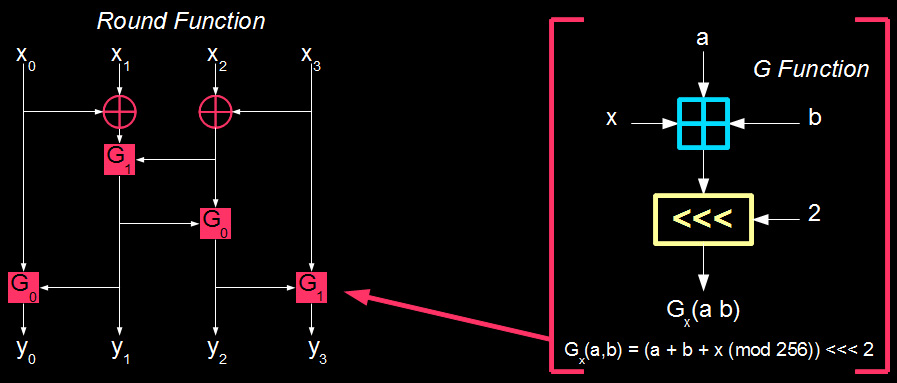The round function itself is pretty easily understood by diagram-staring. The input to it is 32 bits and so is the output. During processing by the round function, everything is split into four 8 bit chunks. These chunks mix together somewhat via XOR operations which provides some diffusion. More diffusion happens in the G function via a cyclic left shift operation that rotates bits around. The modulo addition in the G function provides all of the cipher's confusion. In fact, it is element that needs to behave in a nonlinear way to resist differential cryptanalysis. You can probably guess by now that it isn't successful in that role.

If the round function is the core of FEAL; the G function is the core of the round function. There are really two G functions; one is G0 and other is G1. These can be combined into a Gx function definition but remember that x only ends up equally 0 or 1. All other inputs are outputs for this function are bytes (8 bits). Gx takes two other inputs called a and b. These inputs come from other parts of the round function and need to be combined in a non-linear way (hence the need for the G function. The first step is to add the two inputs together. Next x is added to this total (x can only be a 0 or 1). Then this sum is taken modulo 256. This ensures that the sum does not exceed the size of one byte. Finally, this number undergoes a 2-bit cyclic left shift. In other words, the function shifts every bit two bits to the left. The far left bits wrap around and become the new far right bits. There is no C operation for this cyclic shift; with a little bitwise math, its not hard to write one, though. This shifted number is the output for the G function.

### Differential Basics

Now its time to start building up to the full differential attack on FEAL. We'll start small and work up; for now it would benefit the reader to press the "I believe" button when it comes to how the attack works overall. First we need to build something called a differential characteristic. This is a property that allows an attacker to estimate states inside a cipher without knowing them. Differential cryptanalysis is a chosen-plaintext attack. This means that we are trying to figure out the secret key (or in this case: secret subkeys) by feeding a cipher using that key plaintext and examining the resulting ciphertext.

Ciphers, and specifically their non-linear components like round functions and sboxes, are meant to behave somewhat like pseudorandom number generators. When you feed the non-linear function an input (X), it should produce an output (Y) that resembles the output of a random number generator. An observer should not be able to predict the output given the input via patterns, etc.. Obviously, you can't just have the non-linear function output a random number because its operation must be reproducible. A good non-linear function would take an input X0, change some bits of X0 to produce X1, and the corresponding outputs (Y0 and Y1) should not have a predictable pattern of bit changes. This behavior should extend to how other input properties map to output properties. If we can find a property that maps with a probability other than what a random function would (50% for example), we can exploit it to discover information about the key used in the cipher.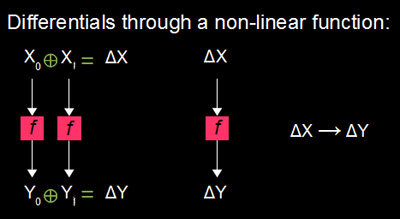The ways in which these patterns map properties of the inputs to properties to the corresponding outputs is called a characteristic also known as a distinguisher. The type of distinguisher that we will be taking advantage of in this attack is called a "differential characteristic". We generate two inputs (X0 and X1) to the target non-linear function that differ by certain bits. This difference is called a differential. To find the differential of two numbers, just XOR them. Next, we feed both of these inputs through the function. Then we take the differential of the resulting outputs (Y0 and Y1). This mapping of input differentials resulting in output differentials is called a differential characteristic.

The probability that any pair of inputs that form a particular input differential leads to a particular output differential should be the same as in a random function. Any deviation from this distribution can be leveraged against the security of the algorithm.

### Behavior of Differentials

Our ultimate goal to predict differentials midway through a cipher by injecting differentials at the plaintext. For this reason, we need to trace how differentials change as they move through the target. We've already seen that when differentials pass through a non-linear function, they are supposed change pseudorandomly. This throws off our tracing efforts completely. However, if a differential passes through the function and changes into a predictable output differential; our tracing efforts can continue. As we'll see soon, one example of high probability differential characteristic that occurs through FEAL's round function is 0x80800000 --> 0x02000000. This characteristic actually occurs with a probability of 1 (100% of the time). Knowing this allows us to tracing a chain of differentials at least partway through the cipher.

So, in our example; we know that when a differential of 0x80800000 is fed into the round function, it produces the output differential 0x02000000. Another property of any non-linear function is that input differentials of 0x00000000 always lead to output differentials of 0x00000000. This makes sense when you think about it because sending data through a function and then sending data with no difference through it will produce the same output (no difference, differential = 0). In other words, sending the same data through the function twice will lead to the same output both times.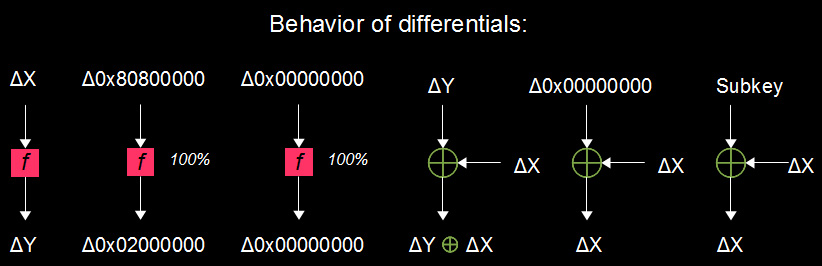Two differentials entering an XOR will perform an XOR operation to produce the resulting differential. This has a handy effect: if identical differentials are XOR'd together, they'll produce an output differential of 0x00000000. Modulo addition is treated like a non-linear function for now, because how it affects differentials is not immediately apparent. Bitwise rotations (cyclic shifts) will shift the bits of differentials as expected.

Key mixing is interesting because it has no effect on the differentials whatsoever. It does effect the actual data but the difference between them. The reason is that the key remains the same even though you are operating on pairs of data. This means that the key input of the mixing XOR operation has a differential of 0x00000000. XORing a differential dX by 0 will always result in the original differential dX.

### Proving our 100% Differential Characteristic

When we start trying to apply differential cryptanalysis to a block cipher, it is necessary to discover a differential characteristic for the round function that holds for a high probability of inputs. Without this, we are dead in the water because the cipher will appear to behave randomly and all of the subkeys will appear equally likely. One way to do this is to run every combination of input pairs through the round function and record how many times each differential characteristic occurs. This works great on small sboxes and function with 4 or even 8 bit inputs/outputs. However, as soon you start looking at larger numbers; this brute force approach becomes unwieldy. Although, all of this calculation is done before the actual attack on the cipher (in the development phase); it will take too long to be feasible.

There exists a 100% characteristic in the FEAL round function such that an input differential of 0x80800000 always leads to an output differential of 0x02000000. We will walk through the round function and discover why this incredibly insecure property exists. The inputs/outputs of the round function are 32 bits long, so brute forcing all of the characteristics is pretty unrealistic (the work is 2^64). So we'll start with the 0x80800000 input differential and trace it through to see why it always leads to 0x02000000.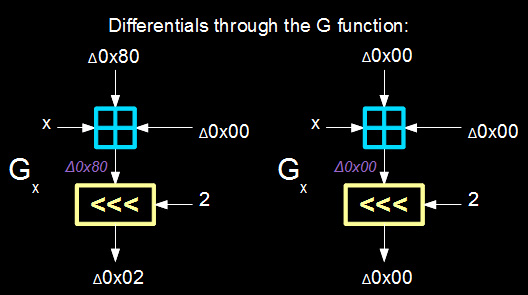The first step is to find some useful characteristics through the G-function. The first obstacle is the addition mod 256. The x input (as in G0 vs. G1) does not affect the path of differentials because it does not change between plaintext encryptions. Thus, the addition of x is treated as a 0 differential. Zero differentials add together to produce a 0 differential in the output of the addition operation. The numbers that form the input differential are still added, but since they are same (zero difference), they produce zero difference in the output. There is another handy characteristic in the addition operation. When a 0x80 differential is fed into it, it produces a 0x80 differential in the output. This 0x80 translates in binary to 10000000b. This means that if only the most-significant-bit changes, then only that same MSB will change in the output. Modulo addition relies on the cascading effect of carrying to make a bit change create other bit changes. Only changing the MSB bypasses this because if there is a carry, the modulo just drops it off the end of the byte and ignores it. This process only works if the other input to the addition operation is differential 0.

The bit rotation just rotates the bits of the differential by 2. This makes 0x80 loop around to become 0x02. Meanwhile 0x00 loops around and becomes 0x00 again. So at the end of the day, the G function has these properties in regard to differentials:

• 0x00 + 0x00 --> 0x00
• 0x80 + 0x00 --> 0x02

### Tracing the Rest of It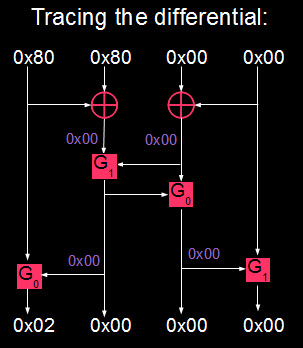Now we'll take what we know about how the G function responds to some differentials and apply it to the full round function of FEAL. We feed it 0x80800000 as the input differential. This 32 bit input is broken up into 4 bytes. The leftmost byte in the diagram is the most-significant byte of the 32 bit integer. We take this "byte 3" and XOR it with byte 2. This output differential is 0 because both input differentials to the XOR operation are the same (0x80). Meanwhile byte 0 and byte 1 XOR to produce a 0 differential. These two zero differentials become the inputs to the first G function (G1). As we established, 0 + 0 leads to yet another 0 output differential. This gives us a final output differential of 0 for byte 2 in the output of the round function. A quick look around will show that byte 1 and byte 0 also become 0 differentials.

The craftiness comes in with byte 3. The zero differential from the first G-function combines with the input byte 3 differential (0x80). We showed earlier that 0x80 leads 0x02 always. This makes the final byte 3 differential output of the round function 0x02. Thus 0x80800000 leads to 0x02000000 in the round function of FEAL.

My god, that was a ton of work just prove that 0x80800000 leads to 0x02000000 in the juicy part of FEAL. Here is what we know about differential characteristics that hold with 100% for the FEAL round function as a whole:

• 0x80800000 --> 0x02000000
• 0x00000000 --> 0x00000000

### Building the Full Path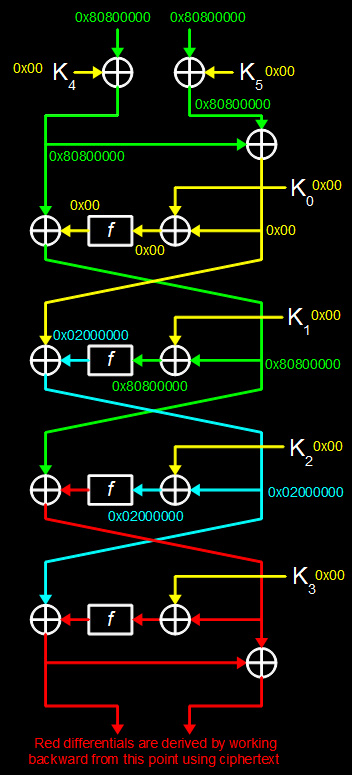So now we've determined some differential properties that hold true for the round function of FEAL. Next, we'll build the actual full differential characteristic using these properties. Our goal is inject an input differential into the plaintext, feed it to the cipher, and trace how the input differential changes with 100% certainty. This gets us about halfway through the 4 rounds. After that uncertainty creeps in. Luckily, we also have differential information from the ciphertext end and can work backwards. This approach is called a meet-in-the-middle attack.

First, we generate 6 pairs of plaintexts with the property that P0 XOR P1 = 0x8080000080800000 and encrypt them. The key/subkeys never change during this process. As a result we get back the corresponding ciphertexts. This style of exploitation is called a "chosen-plaintext attack". If you follow that input differential through the cipher, you'll find a great deal of information about the internal state during encryption. Although you have most of the differentials at various midpoints, you don't know exactly what the individual texts are internally. They are obscured by the key. Luckily for us, key mixing does not alter the differentials. The reason is that the keys stay the same throughout the process; therefore the differential of the key inputs is 0x00.

When the differentials hit a round function, we cannot reliably predict what the output differential will be. There are two exceptions to this rule. When the input is 0x00, the output is 0x00 as well. This is because there is no difference in the inputs, so you are effectively feeding it the same input twice. This produces identical outputs and because the outputs are identical, their difference is 0x00. The other exception is the major flaw in the round function that we found earlier. When 0x80800000 is fed into the round function, the output differential is 0x02000000. We also saw earlier that the XOR operation performs XOR on the differentials (just like it does the actual texts that produce the differentials).

We get as far as the left input to the final round knowing all of the differentials. This path is blocked there by the XOR coming out of the final round function. The reason that we don't know the output differential of the last round function stems from not knowing the output differential of the 3rd round function. This is because the input differential to round 3's function is 0x02000000. We cannot predict reasonably what differential this will produce through the round function.

What we need to figure out is what the output differential of the last round function is. This will be used to test subkeys in the last round later. Luckily, this one is pretty easy to figure out. We find the differential of the left side of the ciphertext. Just XOR the most-significant 4 bytes of one ciphertext (in a pair) with the same bytes of its buddy in that pair. Now check out that XOR that produced that left ciphertext. On one side of it, we know the left ciphertext differential. On the other side we know that the input differential to the left side of the last round is 0x02000000. So we can XOR these two numbers together to find the last round function's output differential.

As far as tracing differentials around, we are finished. We'll do some more backtracing from the ciphertext in moment to find the last round's subkey. That diagram on the left will be your best friend learning how the attack works overall. Studying it while reading code or my feeble attempts at explanation will help greatly.

### Finding the Subkey

Alright, we are finally ready to recover the goodies. It's been a long road to get here and congratulations on making it this far. This stuff is freaking hard, so good job.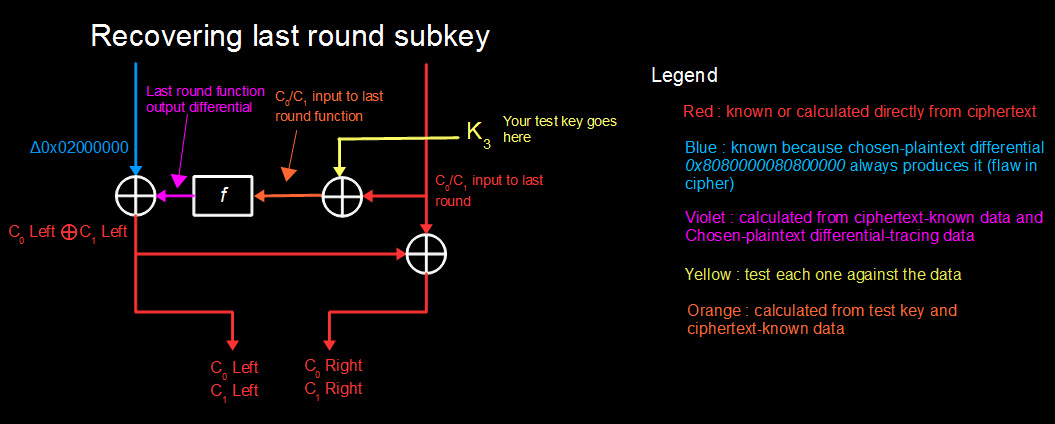We need to figure out the right half (least-significant 4 bytes) of the input to the last round. NOT THE DIFFERENTIAL. We need to find the actual pair of texts that go into the last round function. We don't care what their difference is. Now we saw earlier that key mixing makes it impossible to trace the texts from the chosen plaintexts. So instead we'll grab them from the resulting ciphertext. Do the following for each ciphertext in a pair:

Perform an XOR on the left ciphertext against the right ciphertext. This gives you the right input texts to the last round. Now do the following for each possible subkey. Start from 0x00000000 and iterate all the way through to 0xFFFFFFFF. On each iteration, XOR this candidate subkey with the round 4 right input that you just found. The result of this operation is the pair of input texts that went into the last round function during encryption.

So, run these texts individually through the round function to produce a pair of last round output texts. XOR these together to get a candidate differential. If this matches the real last round function output differential that you calculated earlier, it means that the candidate key you used is a good bet for being the real one. One way to figure out what the real subkey is: keep score for each candidate subkey and increment the score if these differentials match. Do this for every pair of chosen-plaintext/ciphertext and keep upping the counts are needed. In the end, you should have a small handful of subkeys that score the highest. In some cases, one of them will have a higher score than all of the others. This is the last round's subkey.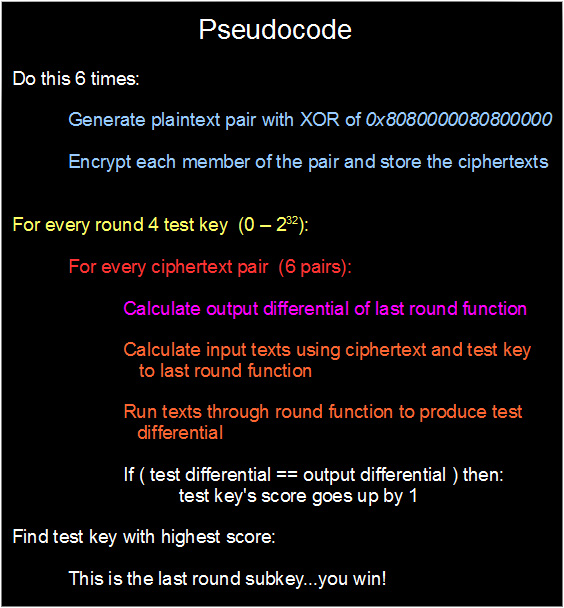### Cracking the Remaining Rounds

Now we'll discuss the way forward toward cracking the full key (actually 192 bits of subkey material). The big gotcha with this process is that you cannot just reuse the above differential path to crack rounds 2 and 3. You must build new differential characteristics that only cover the uncracked rounds. Before you use these new characteristics, you must decrypt the last round using the subkey found via the technique above. Once you've decrypted the last round, you cam repeat the process of guessing and testing subkeys by matching the predicted output differential.

Luckily for us, it is easy to build these new shorter differential paths. We simply create them by trimming down the original differential path from the input end. This means that all of our differential paths for rounds 2-4 will have the same output differential but different input differentials. This also means that we'll need to generate new chosen-plaintext pairs for each round using these input differentials. Here are the differentials that I used for each of the rounds:

• Round 4: 0x8080000080800000-->0x02000000
• Round 3: 0x0000000080800000-->0x02000000
• Round 2: 0x0000000002000000-->0x02000000

Now you're probably wondering how we'll crack round #1. The first round is tricky because we can't use differentials to get its subkey. It also has two more subkeys that mix in right before it. Our goal is to recover all three of these subkeys in one shot. To do this, guess K0 and decrypt through round 1 like usual. Next, use that along with a chosen-plaintext pair to calculate K4 and K5. Repeat this process and keep track of which K0 guesses lead to identical K4s and K5s with every chosen-plaintext pair. The K0 guess that keeps the corresponding K4 and K5 consistent is the correct K0 (this also gives us K4 and K5). Now we have the full 192 bits of subkey material!

### Power of the Attack

Exhaustive search of the keyspace from the perspective of subkeys means brute forcing every permutation of 192 bits. This comes out to testing approximately the following number of keys:

6,300,000,000,000,000,000,000,000,000,000,000,000,000,000,000,000,000,000,000

That's ridiculous. By finding the subkeys our way, we end up testing about 171,798,691,840 keys. We also do far less work because most of our calculations are done inside 1 round rather than the full cipher. The process takes about 90 seconds on my laptop without any fancy optimization tricks (beyond just turning on optimization in the compiler).